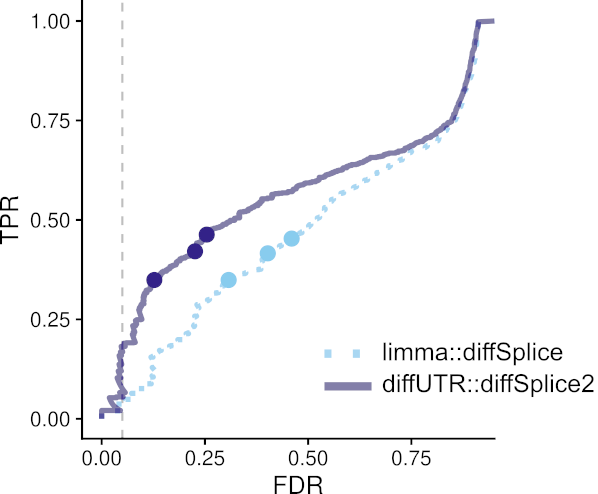Here we describe the improvements to limma’s diffSplice method. diffSplice works on the bin-wise coefficient of the linear model which corresponds to the log2 fold changes between conditions. It compares the log2(fold change) $$\hat{\beta}_{k,g}$$ of a bin $$k$$ belonging to gene $$g$$, to a weighted average of log2(fold change) of all the other bins of the same gene combined $$\hat{B}_{k,g}$$ (the subscript $$g$$ will be henceforth omitted for ease of reading). The weighted average of all the other bins in the same gene is calculated by $\begin{equation} \hat{B}_{k}= \frac{\sum_{i, i\neq k}^{N}{w_{i} \hat{\beta}_{i}}}{\sum_{i, i\neq k}^{N}w_{i}} \end{equation}$ where $$w_{i}=\frac{1}{u_{i}^2}$$ and $$u_{i}$$ refers to the diagonal elements of the unscaled covariance matrix $$(X^TVX)^{-1}$$. $$X$$ is the design matrix and $$V$$ corresponds to the weight matrix estimated by voom. The difference of log2 fold changes, which is also the coefficient returned by diffSplice() is then calculated by $$\hat{C}_{k} = \hat{\beta}_{k} - \hat{B}_{k}$$. Instead of calculating the t-statistic with $$\hat{C}_{k}$$, this value is scaled again in the original code:

$\begin{equation} \hat{D}_{k} =\hat{C}_{k}\sqrt{1-\frac{{w}_{k}}{\sum_{i}^{N}w_{i}}} \end{equation}$

and the t-statistic is calculated as:

$\begin{equation} t_{k} =\frac{\hat{D}_{k}}{u_{k}s_{g}} \end{equation}$

$$s_{g}^2$$ refers to the posterior residual variance of gene $$g$$, which is calculated by averaging the sample values of the residual variances of all the bins in the gene, and then squeezing these residual variances of all genes using empirical Bayes method. This assumes that the residual variance is constant across all bins of the same gene.

In , we applied three changes to the above method. First, the residual variances are not assumed to be constant across all bins of the same gene. This results in the sample values of the residual variances of every bin now being squeezed using empirical Bayes method, resulting in posterior variances $$s_{i}^2$$ for every individual bin $$i$$. Second, the weights $$w_{i}$$, used to calculate $$\hat{B}_{k}$$, now incorporate the individual variances by $$w_{i}=\frac{1}{s_{i}^2u_{i}^2}$$. Third, the $$\hat{C}_{k}$$ value is directly used to calculate the t-statistic, which after all these changes now corresponds to

$\begin{equation} t_{k} =\frac{\hat{C}_{k}}{u_{k}s_{i}}. \end{equation}$

Using a simulation from a previous DEU benchmark Soneson et al. 2016, we show that our modifications improve the accuracy of the original limma method:Figure 1: diffSplice2 improves upon the original method in a DEU simulation

Of note, however, in most settings the accuracy of diffSplice2 is still inferior to that of DEXSeq, which therefore remains the method of choice for DEU. However, as the computationally-heavy DEXSeq does not scale well and is not applicable in all situations, we recommend diffSplice2 as the next best method.